• matlab中gui界面设计
千次阅读
2021-04-18 06:47:57

最近使用Matlab中的GUI模块进行了界面设计，其中有一些体会，写出来以便以后使用。

闲话少说，介绍一下我遇到的问题以及解决方案：

1、文本框输入的数据的采集和计算输出。

错误提示：Undefined

function or variable "a".

每一个数据框下的callback函数后加上下面的语句，不要有任何改动。(帖子里有介绍)

% handles

包含figure中所有图形对象句柄的结构体，如果我们想引用tag为mytag的控件，使用handles.mytag

% set/get 函数是用来设置/获取某个控件属性

% 使用get命令获取第一个加数，并将它转换成数值

input = str2num(get(hObject,'String')); %

string属性是字符串，所以必须转换成数值

% 检验输入是否为空，是则将它置为0

if (isempty(input))

set(hObject,'String','0')

end

%

保存handles结构体，只要在handles结构体有改变时，才需要保存

guidata(hObject, handles); %

这里由于handles没有改变，故这里其实没有必要，但是为了避免潜在的不必要麻烦，建议在所有Callback最后都添加该命令。

**********************

在pushbutton的callback函数中插入下面的语句：(这些语句可以改动，但标色部分要存在。)

a = get(handles.input1_editText,'String');

b = get(handles.input2_editText,'String');

% a和b是字符串变量，我们需要使用str2double函数将其转换为数值

% 然后才能相加，否则字符串是没法相加的

total = str2num(a) +

str2num(b); % 格式转换，转换为数值

%这次之后就获得了数字，可以随意操作。

% 由于string属性是字符串，所以必须将两个数的和转换为字符串

c = num2str(total); % 转换为字符串

guidata(hObject, handles); % 更新结构体

2、找不到已经删除的函数

错误提示：Undefined

command/function 'hanshu_CreateFcn'.

打开GUI界面重新重新编辑属性框，把属性框中的callback和createfcn两栏后面的名字改正就ok了。

3、某些计算的中间变量在command window里出现。

计算变量后忘记加“;”了，但这种错误并不影响计算结果。

其中第一个问题比较重要，希望大家重视。但是一定这样处理的原因不了解，希望知道的大神给予解决~更多相关内容
• 我所使用的MATLAB版本——MATLAB R2017a MATLAB的GUI的操作其他人写的很清楚了，在此不再赘述。 MATLAB的GUI的基本操作可见：GUI基本操作 这次所设计的钢琴有简单的七个琴键，DO RE ...其中的STRING代表在GUI界面的名字
• MATLAB GUI界面设计，简单易懂，GUI设计比较好，建议学习。
• 通过输入焊缝坡口某界面的4个特征点，可自行生成焊缝坡口的理想三维模型，适合初学matlab gui的道友
• 利用MATLAB中GUI工具来制作串口通信上位机，利用上位机将数据利用绘图实时显示出来。
• Matlab实现语音分析：加噪声，频谱分析，滤波器等等matlab设计一个语音输入GUI界面，可以添加噪声,单频噪声,多频噪声,设置IIR滤波或者FIR滤波+含代码操作演示视频 运行注意事项：使用matlab2021a或者更高版本测试，...
• 大三上小学期学习了MATLABGUI界面设计，感觉挺不错的，这里做个总结！ 基本流程 1、命令行输入“guide”指令，打开GUI面板，选择“新建GUI”、“Blank GUI” （左侧工具栏含有待添加的元素） 2、添加...

大三上小学期学习了MATLAB的GUI界面设计，感觉挺不错的，这里做个总结！

# 基本流程

1、命令行输入“guide”指令，打开GUI面板，选择“新建GUI”、“Blank GUI”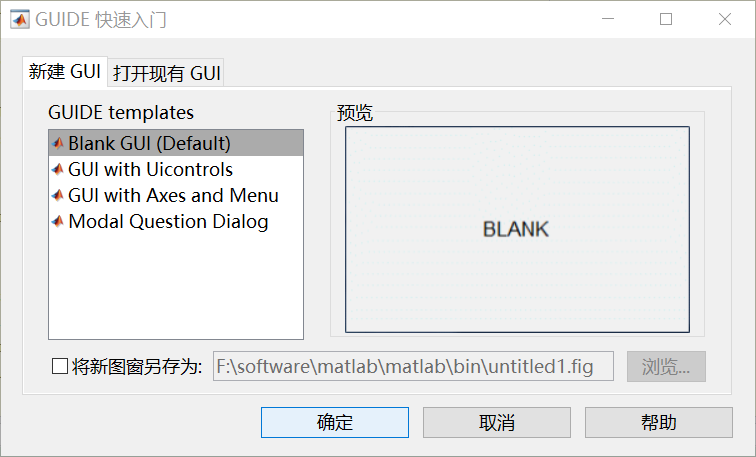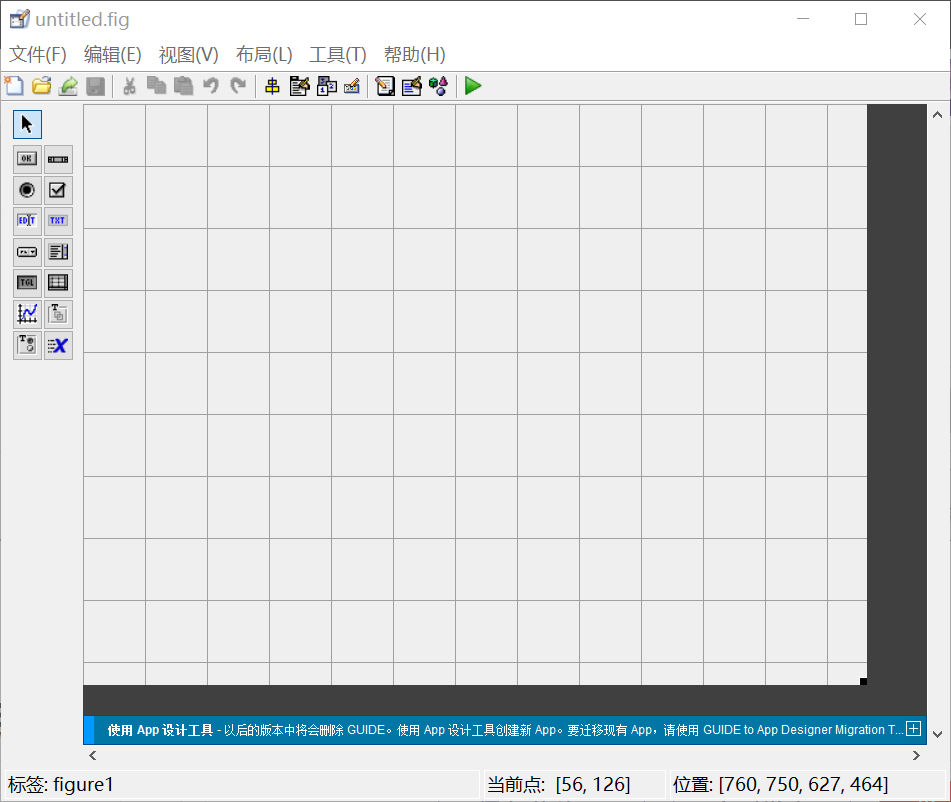（左侧工具栏含有待添加的元素）

2、添加所需要的的元素（一般需要普通按钮、静态文本、普通文本、坐标图、单选按钮等）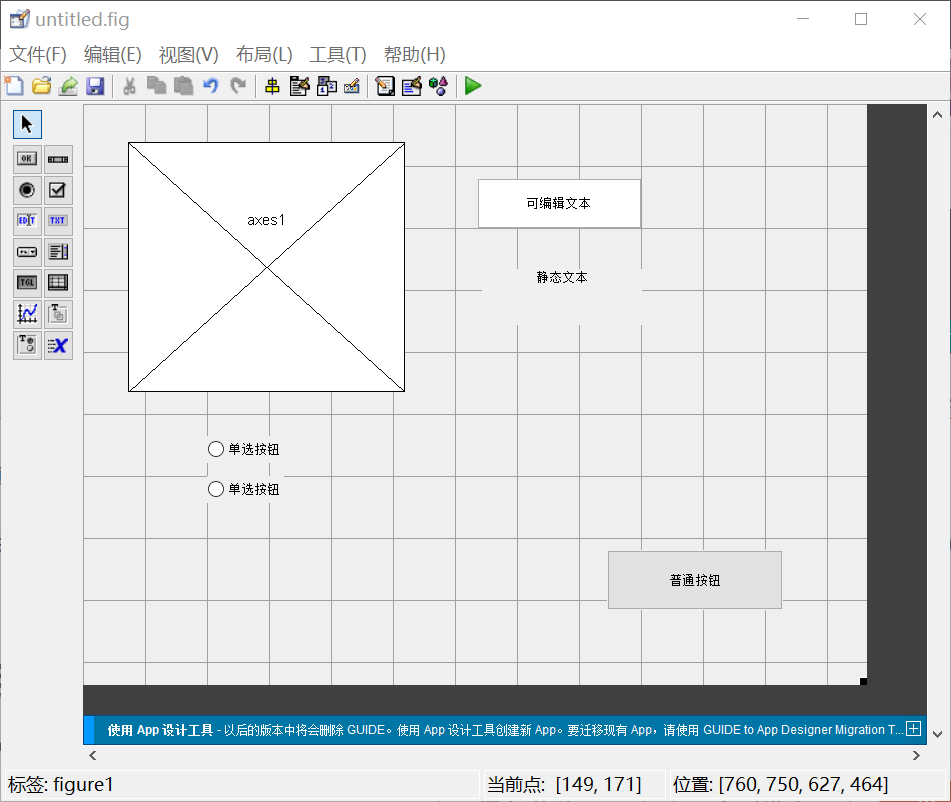3、右键“普通按钮”，选择“查看回调”中的“CALLBACK”，输入对应的MATLAB命令（可以看做编写函数，点击按钮是进行调用）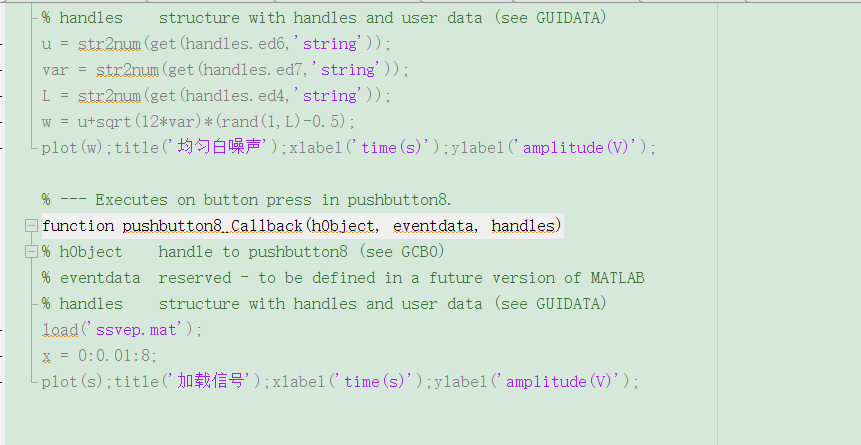（此图仅用于举例，代码不全）

4、运行调试

# 常用代码

1、将动态文本（可编辑文本）的值赋予代码中某变量（以变量A为例）

A = str2num(get(handles.ed1,'string'))；

（代码中“ed1”是可编辑文本的标签，在GUI界面可设置）

2、确定坐标图

因为一个GUI中可能设置多个坐标图，所以也需要标签（Tag）确定

axes(handles.axes1)；

上图中“axes1”是设置的标签

3、赋予动态文本值

（这个是方便将计算结果展示出来用的）

set(handles.ed1,'string',x);



ed1是动态文本的标签，x是变量名字，相当于把x这个变量的值显示在动态文本里

# 元素介绍

以动态文本为例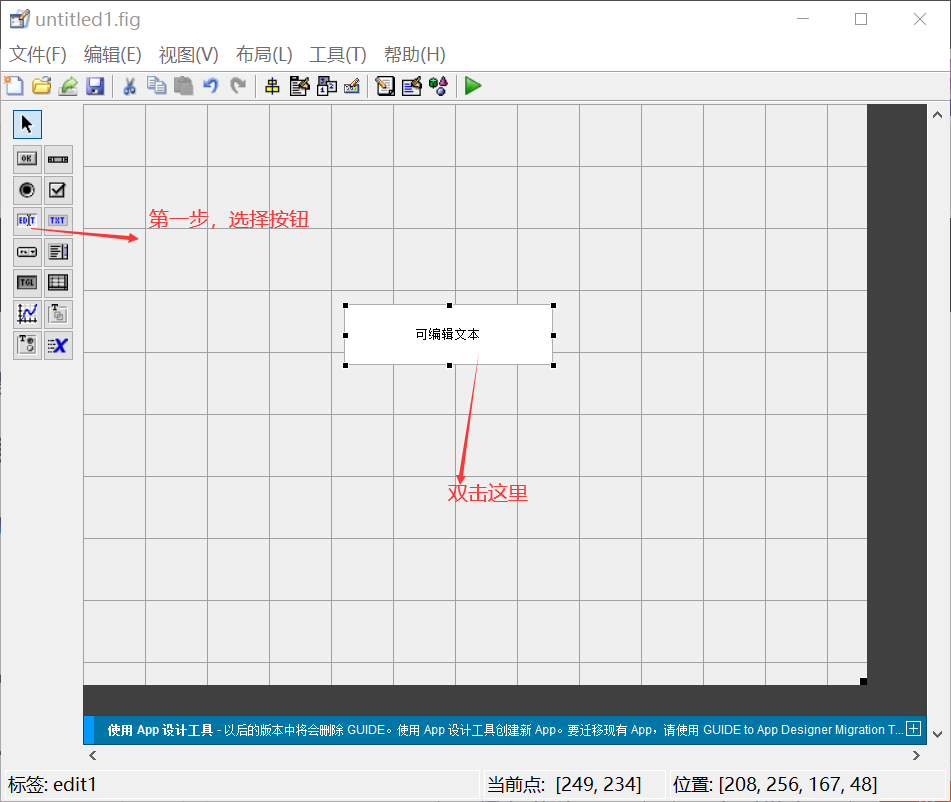双击后效果如下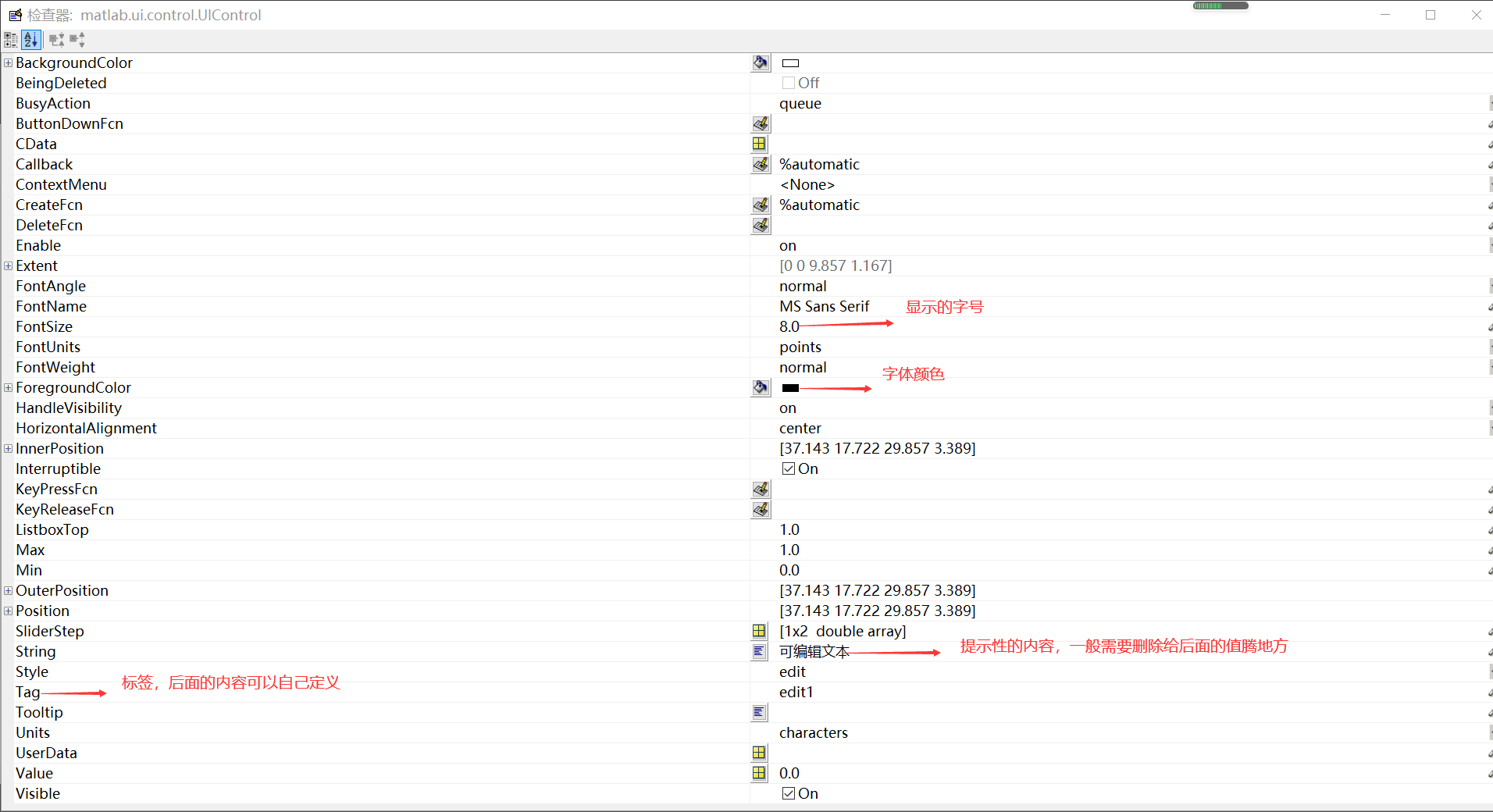# 举个栗子

就拿绘制为例吧

第一步，用元素构造GUI界面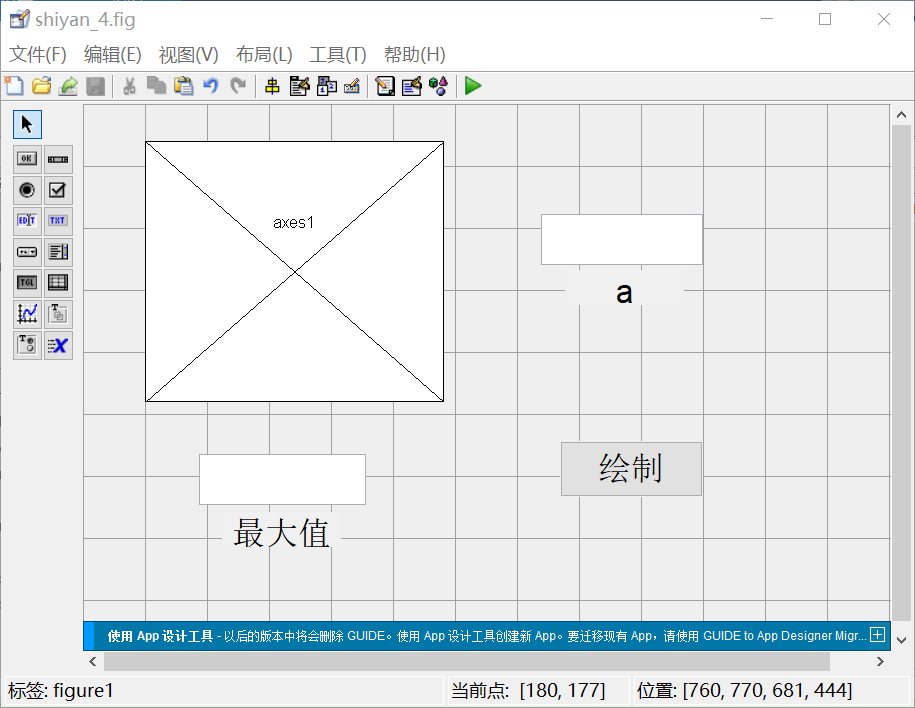第二步，运行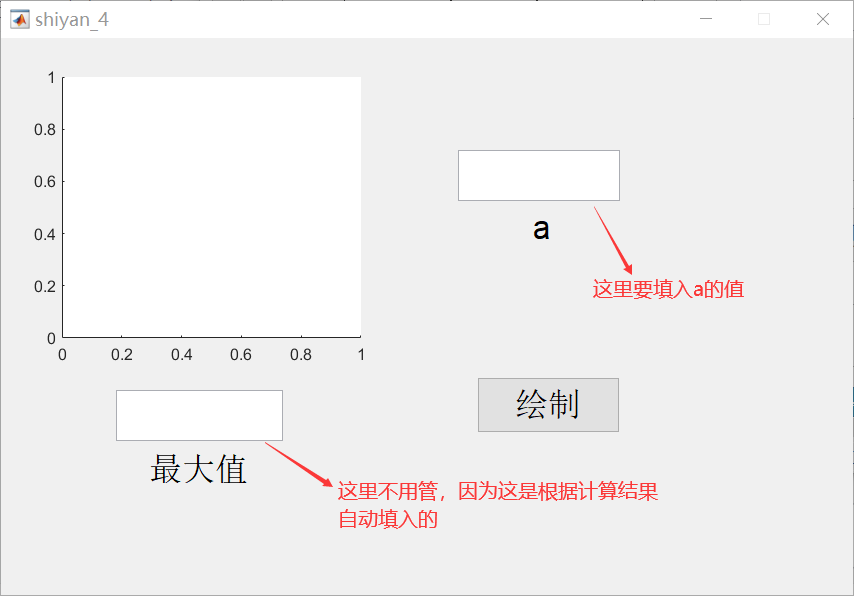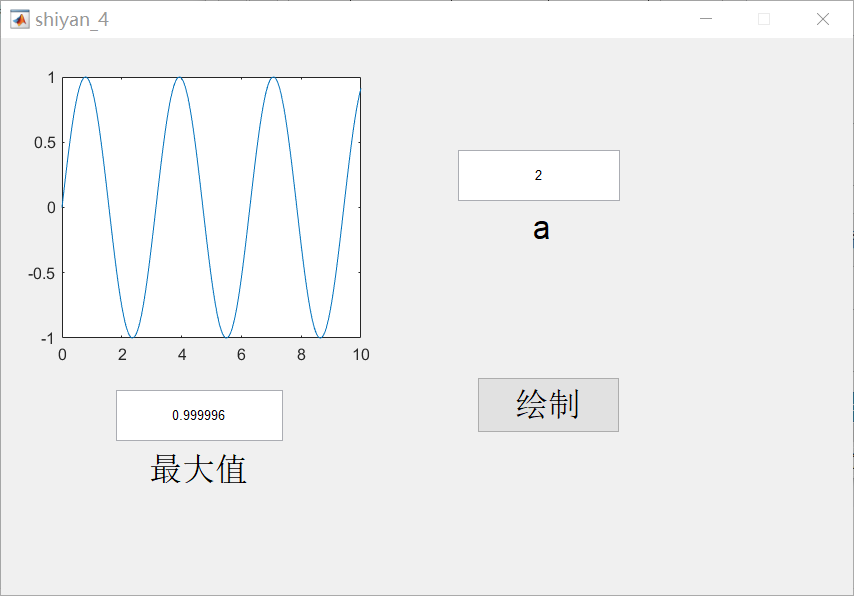3、参考代码

function pushbutton4_Callback(hObject, eventdata, handles)
% hObject    handle to pushbutton4 (see GCBO)
% eventdata  reserved - to be defined in a future version of MATLAB
% handles    structure with handles and user data (see GUIDATA)
A = str2num(get(handles.ed1,'string'));
x = [0:0.01:10];
y = sin(A.*x);
set(handles.ed2,'string',max(y));
axes(handles.axes1);
plot(x,y);

### 欢迎大家批评指正！后续随着学习深入会继续更新！

展开全文• 软件界面上能显示课程设计题目、四则运算控制按钮、两组输入控件、一组输出控件，可实现连续运算，可实现单个元素的运算，也可实现一组数据的运算，可显视运算成功或运算错误提示。
• MATLAB实现linear;pchip;spline三种不同插值方法的GUI界面设计
• 1. matlabGUI界面设计简单介绍 一个很好的界面能实现机交互，好的UI设计不仅是让软件变得有个性有品位，还要让软件的操作变得舒适简单、自由，充分体现软件的定位和特点。 matlab有它自己的界面设计模块——...

# 1. matlab的GUI界面设计简单介绍

一个很好的界面能实现机交互，好的UI设计不仅是让软件变得有个性有品位，还要让软件的操作变得舒适简单、自由，充分体现软件的定位和特点。
matlab有它自己的界面设计模块——“GUIDE”

GUI（Graphical User Interface，GUI）指的是图形用户界面，也称之为图形用户接口，GUI是用图形化方式显示的计算机操作用户界面（可视化）。

# 2. GUI界面的入门操作——数据传递

如何打开GUI设计界面
通常在matlab 的命令窗口输入“guide”指令，即可得到：首先我们来简单实现数据传递的功能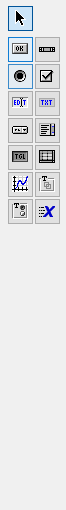在左侧功能框中，选择“按钮”并在设计框中画出按钮的大小
再选择“可编辑文本”，设计框中添加两个可编辑文本
我们的想法很简单，就是当我们按下按钮时，实现其中一个可编辑文本的内容出现在另一个可编辑文本中，实现数据的传递。
点击可编辑文本，记住它的“Tag”，之后我们要实现对其调用，都是通过Tag来实现的，同时同时，我们把“String”文本的内容清零。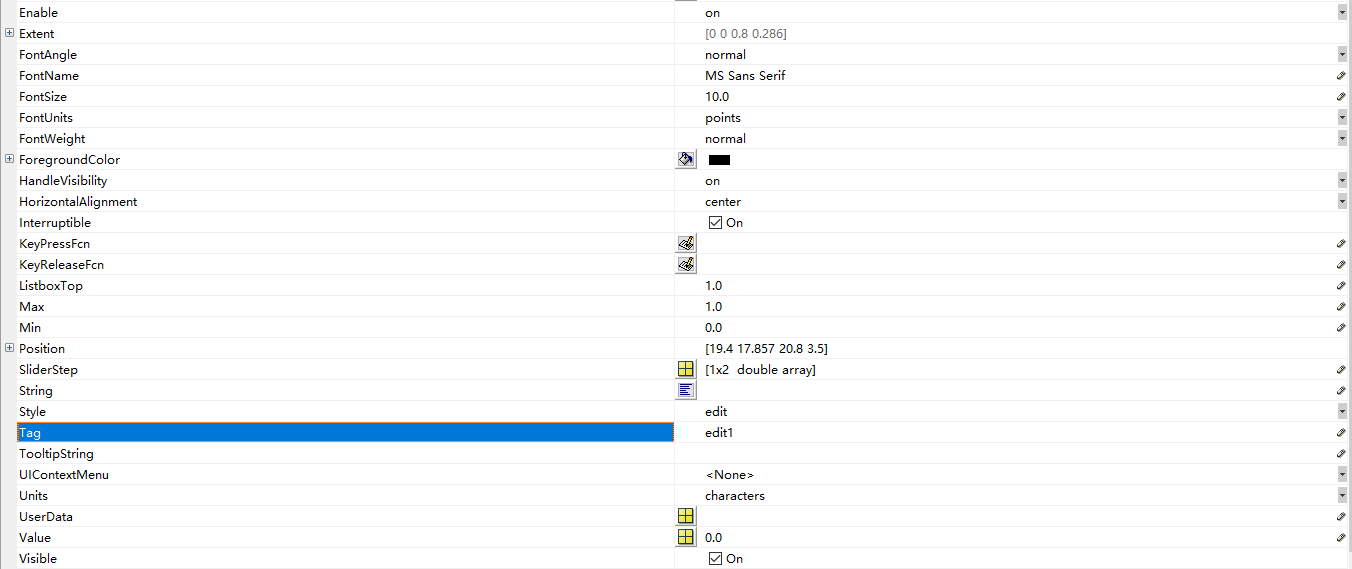然后，我们左击“按钮”，把“String”按钮的文本内容改为“数据传递”】我们点击按钮的操作实际上是对按钮的回调函数进行的调用，因此，我们右击“按钮”，在“查看调用”中，选择“Callback
我们写这样的代码：

str=get(handles.edit1,'String');
set(handles.edit2,'String',str);

第一行代码：首先获取第一个“可编辑文本”edit1的文本内容，将它存储在变量str中
第二行代码：将变量str的内容写入**“可编辑文本**”edit2中

handles是一个以GUI中所有控件的Tag属性为字段的结构体，每个字段的取值就是对应控件的句柄！
也就是说：所有控件的Tag都是结构体handles的唯一存在元素，通过Tag，我们找到具体的对象，实现对该对象的内容的操作。

之后，我们再讲“GUI界面之滚动条”

展开全文• 点击signal_generator.fig即可运行整个程序，代码在matlab R2018b上绝对完美运行，GUI纯小白建议预习再下，如果不会打开GUIDE，百度一下再打开，不要报了bug就说代码不能运行。
• matlab中GUI界面的一些设计与操作

# GUI界面建立

成果：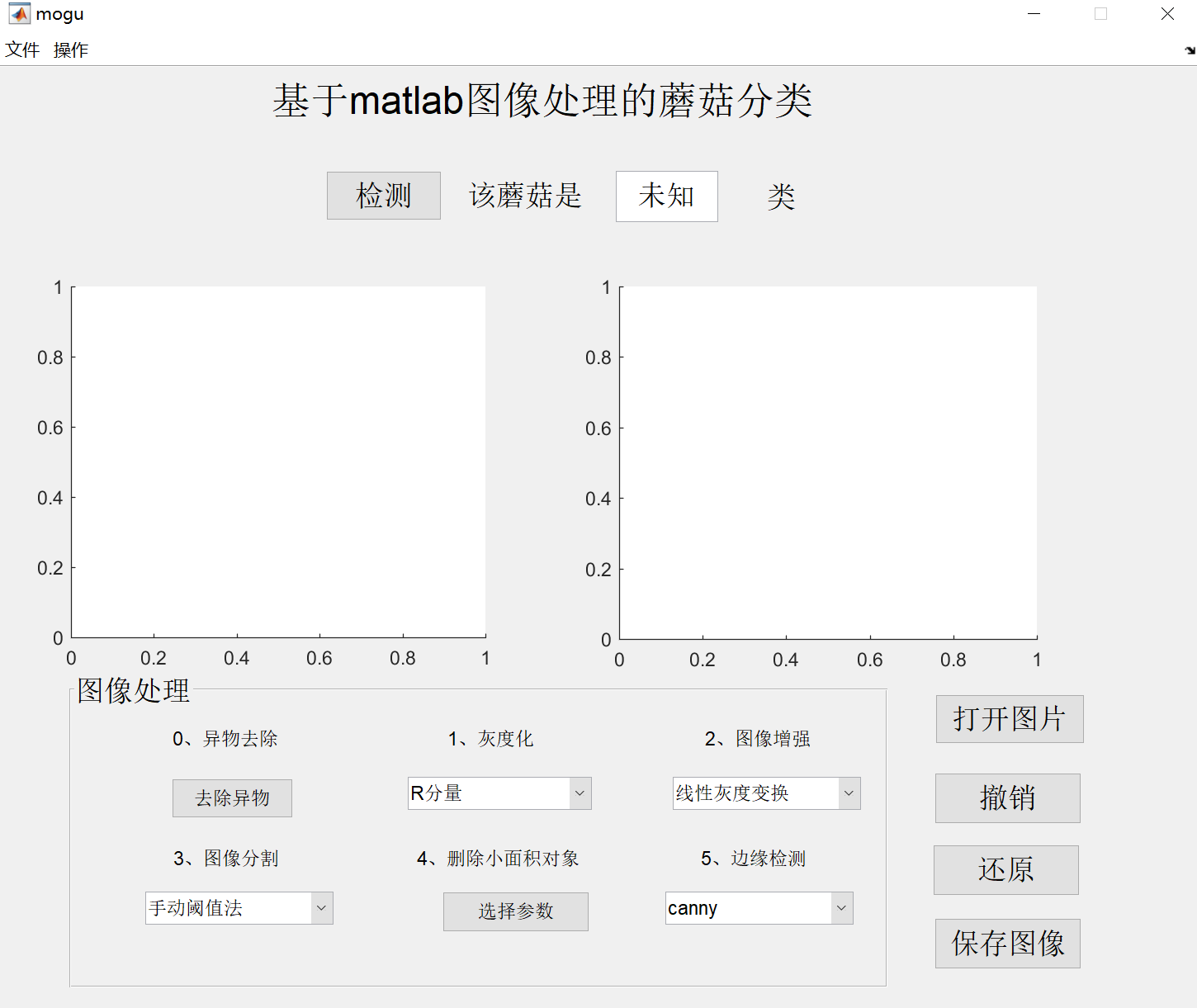在这里只介绍其中几个按钮的作用，不一一赘述，有问题可以私信，之后也会上传完整代码

## （1）新建## （2）设计页面

先设计页面，再编写回调函数！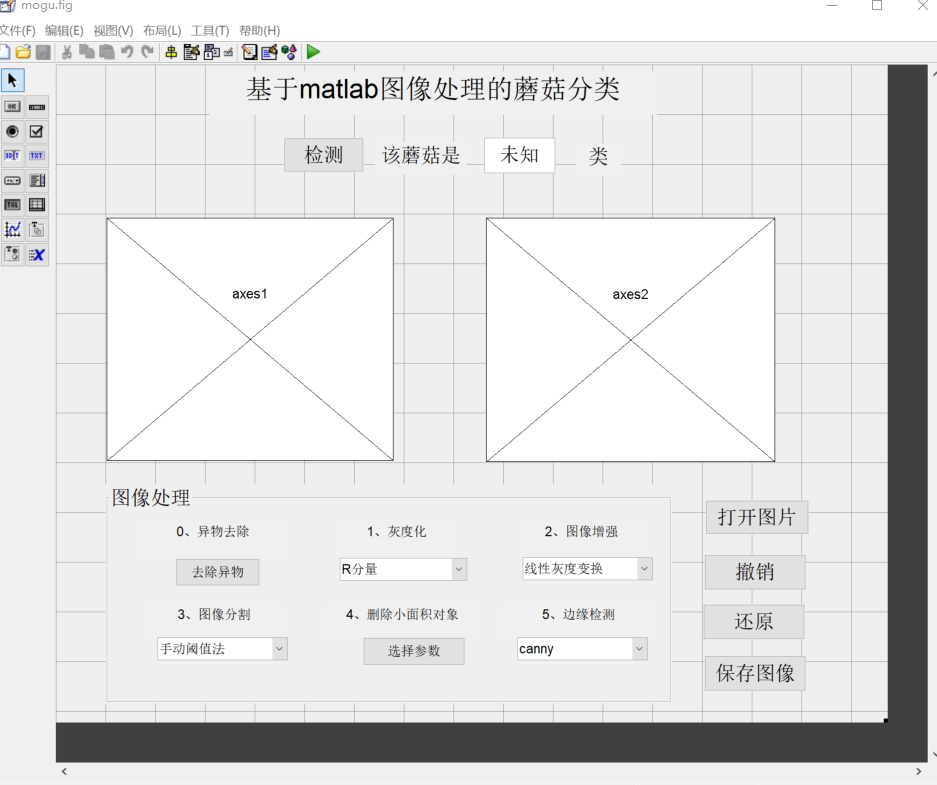一般需要更改大小、文字，最重要的是要编辑tag值，以便找到回调函数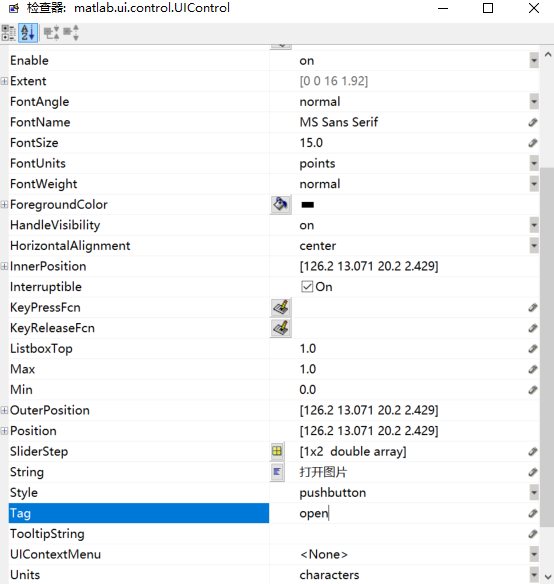鼠标右键点击按钮，选择查看回调函数callback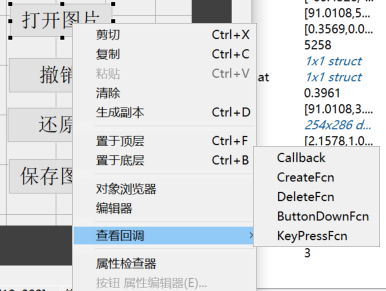在回调函数中编辑，点击后的反应

### a、打开图片按钮

function open_Callback(hObject, eventdata, handles)
% hObject    handle to open (see GCBO)
% eventdata  reserved - to be defined in a future version of MATLAB
% handles    structure with handles and user data (see GUIDATA)
% axis off  %%关闭坐标轴显示
global IMG0;
global IMG1;
global IMG2;
global IMG3;
global IMG4;
IMG0=0;
IMG1=0;
IMG2=0;
IMG3=0;
IMG4=0;
[filename pathname] =uigetfile({'*.*';'*.png';'*.JPEG';'.jpg';'*.bmp'},'打开图片');
if isequal(filename,0)||isequal(pathname,0)
msgbox('没有选中文件','出错');
return;
else
file=[pathname filename];
%%打开图像
%%打开axes1的句柄 进行axes1的操作
axes(handles.axes1);
axis tight;
%%在axes1中显示图像
imshow(RGB);
title('原始图像');
%% ****************************剪裁图像********************************
r=RGB(:,:,1);
% I=mat2gray(r);%实现图像矩阵的归一化，使矩阵的每个元素的值都在0和1之间
%I = rgb2gr11ay(RGB);%彩色图像转灰度图像
%% *******************图像分割（阈值法）*******************
%最大类间方差法自动计算灰度图像I阈值，再用im2bw得到二值图像
threshold = graythresh(r);
bw = im2bw(r,threshold);
% figure,imshow(bw)
%% ***************************形态学处理*********************
%删除二值图像bw中面积小于150的对象，默认情况下使用8邻域。
bw = bwareaopen(bw,30);
%闭合（先膨胀后腐蚀），填充物体内细小空洞，连接邻近物体，平滑边界
se = strel('disk',5);
bw = imclose(bw,se);
% 填充二值图像中的由边界包围的空洞区域。
bw = imfill(bw,'holes');
% figure,imshow(bw)
%% *******************************以上是图像二值化，下面找邻接矩阵*******************************
% 返回一个和bw大小相同的L矩阵，包含了标记了bw中每个连通区域的类别标签，这些标签的值为1、2、num（连通区域的个数），默认为8连通寻找
[L,n] = bwlabel(bw);
x=zeros(1,n);
for i=1:n
x(i)=sum(sum(L==i));
end
[m,ind]=max(x);
[y,x]=find(L==ind);
x0=min(x(:));%找连通域列中最小值
x1=max(x(:));
y0=min(y(:));
y1=max(y(:));
hold on
% rectangle('Position',[x0,y0,x1-x0,y1-y0],'edgeColor','g','LineWidth',1)%标出邻接矩阵
I = imcrop(RGB,[x0,y0,x1-x0,y1-y0]);
% figure,imshow(I3);
axes(handles.axes2);
imshow(I);
global name;
name='剪裁后的图像';
title(name);
global S;
S=I;%设置全局变量，保存初始图像路径，以便还原
global K;
K=1;
global N;
N={};
N{K}=name;
global B;
B={};
B{K}=I;
IMG0=I;
end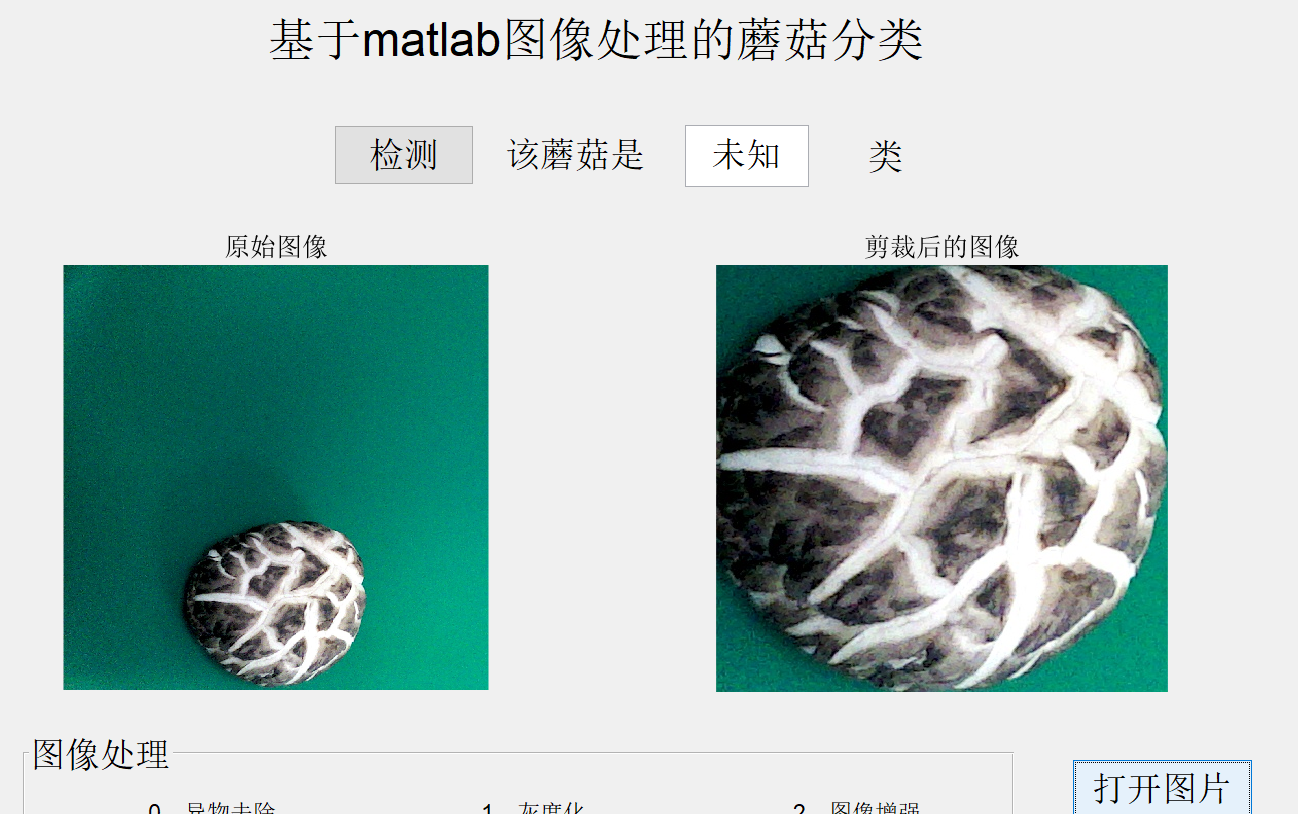### b、选择参数按钮

对于这个选择参数跳出方框的设计：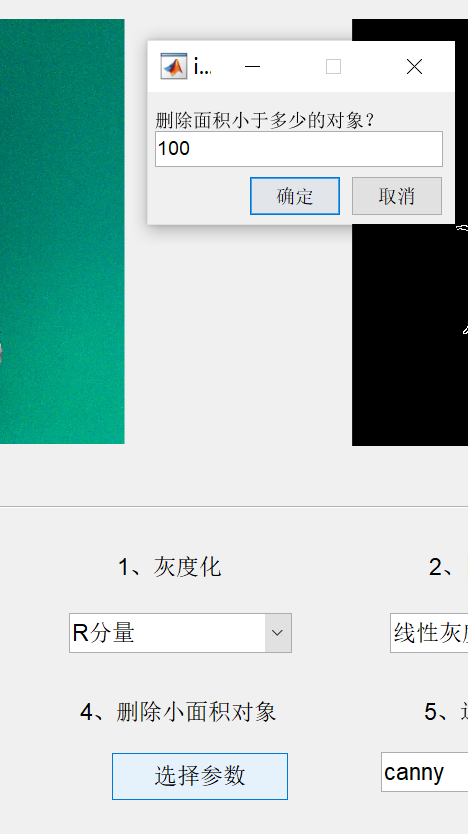设计页面中双击按钮，可在检查器中设计显示中文"选择参数"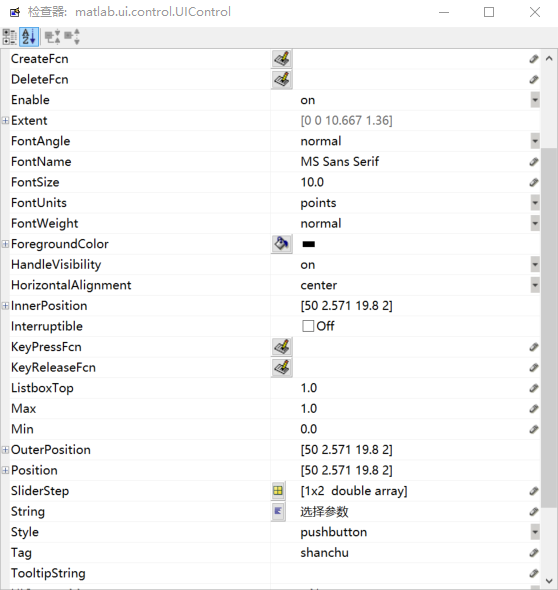% --- Executes on button press in shanchu.
function shanchu_Callback(hObject, eventdata, handles)
% hObject    handle to shanchu (see GCBO)
% eventdata  reserved - to be defined in a future version of MATLAB
% handles    structure with handles and user data (see GUIDATA)
global B;
global name
global N;
global K;
global IMG3;
global IMG4;
global IMG0;
if IMG0==0
msgbox('无图片');
elseif IMG3==0
msgbox('请执行上一步');
else
I=IMG3;
axes(handles.axes2);
prompt={'删除面积小于多少的对象？'};
defans={'100'};%设置默认值
p=inputdlg(prompt,'input',1,defans);
p1=str2num(p{1});
I1=bwareaopen(I,p1);
guidata(hObject,handles);
imshow(I1);
name='手动阈值法';
title(name);
K=K+1;
B{K}=getimage(handles.axes2);
N{K}=name;
IMG4=B{K};
end


### c、多项选择按钮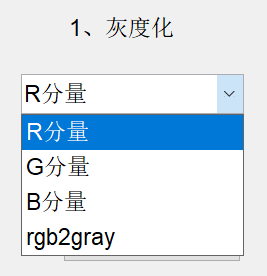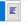里面填上选项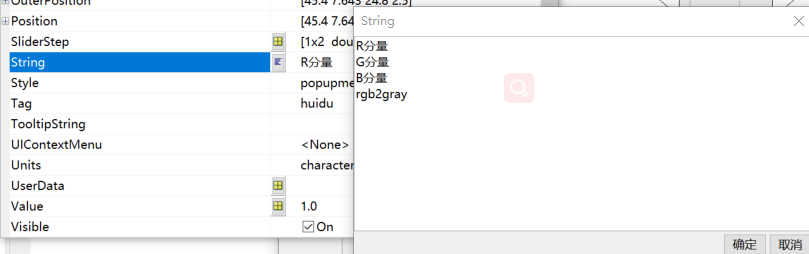在回调函数（huidu_Callback）里对var参数进行判断

% --- Executes on selection change in huidu.
function huidu_Callback(hObject, eventdata, handles)
% hObject    handle to huidu (see GCBO)
% eventdata  reserved - to be defined in a future version of MATLAB
% handles    structure with handles and user data (see GUIDATA)

% Hints: contents = cellstr(get(hObject,'String')) returns huidu contents as cell array
%        contents{get(hObject,'Value')} returns selected item from huidu
global B;
global name;
global N;
global K;
global IMG0;
global IMG1;
if IMG0==0
msgbox('无图片');
IMG1=0;
else
I=IMG0;
var=get(handles.huidu,'value');
axes(handles.axes2);
I=mat2gray(I);
switch var
case 1
I1=I(:,:,1);%图像增强
imshow(I1);
name='取R分量后的图像';
title(name);
case 2
I1=I(:,:,2);%图像增强
imshow(I1);
name='取G分量后的图像';
title(name);
case 3
I1=I(:,:,3);%图像增强
imshow(I1);
name='取B分量后的图像';
title(name);
case 4
I1=rgb2gray(I);%图像增强
imshow(I1);
name='取B分量后的图像';
title(name);
end
K=K+1;
B{K}=getimage(handles.axes2);
N{K}=name;
IMG1=B{K};
end


### d、检测按钮

单击检测按钮会在后面的方框中出现检测结果：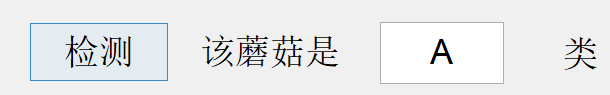利用set(handles.edit1,‘string’,a);函数设置，后面的方框名字是handles.edit1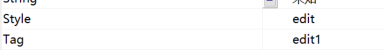% --- Executes on button press in jiance.
function jiance_Callback(hObject, eventdata, handles)
% hObject    handle to jiance (see GCBO)
% eventdata  reserved - to be defined in a future version of MATLAB
% handles    structure with handles and user data (see GUIDATA)
global IMG0
if IMG0==0
msgbox('无图片');
else
I = IMG0;
R=I(:,:,1);
I1=mat2gray(R);%实现图像矩阵的归一化，使矩阵的每个元素的值都在0和1之间
%% 图像增强—线性灰度变换
%% 手动阈值法图像分割
k1=find(I1>180/255);     %找到灰度值大于180的索引（指针）
I2=zeros(size(I1));
I2(k1)=255/255;  %索引大于180的像素的灰度值置255
%% 删除面积小于100的对象
bw = bwareaopen(I2,100);
%% 边缘检测—canny算子
ed=edge(bw,'canny');
sum1=0;
[M,N]=size(ed);
for i=1:M
for j=1:N
if ed(i,j)==1
sum1=sum1+1;
end
end
end
n=sum1/(M*N);
%% ************计算最大连通域面积*********
[M,N]=size(bw);
[L,n] = bwlabel(bw);
x=zeros(1,n);
for k=1:n
x(k)=sum(sum(L==k));
end
[m,ind]=max(x);
con=0;
S=zeros(M,N);
for k=1:M
for j=1:N
if L(k,j)==ind
con=con+1;
S(k,j)=1;
end
end
end
CON=con/(M*N);
%% **********************************特征选择*****************************
h=imhist(R); %用红色分量图最好，灰度图次之，二值图再次之，边缘图方差很近
h=h./sum(h);  %归一化
L=length(h); %计算灰度级
L=L-1;
h=h(:);      %转化为列向量
stm=zeros(1,3);
stm(1)=m;
for j=2:3
end
usm(1)=stm(1)*L;%一阶矩
usm(2)=stm(2)*L^2;%二阶矩
%usm(3)=stm(3)*L^3;%三阶矩
st(1)=usm(1); %均值
st(2)=usm(2).^0.5; %标准差
%st(3)=1-1/(1+usm(2)); %平滑度
st(3)=sum(h.^2); %一致性
%st(5)=-sum(h.*log2(h+eps)); %熵
%% **********模型*******
svmModel= svmModel_mat.svmModel;
%计算超平面方程参数
[~, r] = size(svmModel.SupportVectors);
w = sum(svmModel.SupportVectors .* repmat(svmModel.Alpha, 1, r));
b = svmModel.Bias;
%将需要验证的样本预处理, （缩放平移）
scale = svmModel.ScaleData.scaleFactor;
shift = svmModel.ScaleData.shift;
%获取样本
sData=[st(2),CON];      %本预测集只有这一个点，输出classes=-0.5918小于零，是线下类（B类蘑菇）
[c, ~] = size(sData);%获取样本数量, sData是样本 每一行是一个样本
sDataScaled = (sData+ repmat(shift, c, 1)) .* repmat(scale, c, 1);
%计算样本的类别
classes = sDataScaled * w' + b;
%算出来的classes小于0的是正类， 大于0的是负类
if classes>0
a='A';
else
a='B';
end
set(handles.edit1,'string',a);
end


## （3）菜单栏设计

对于右上角的菜单栏设计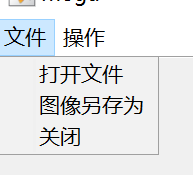点击菜单编辑器进行编辑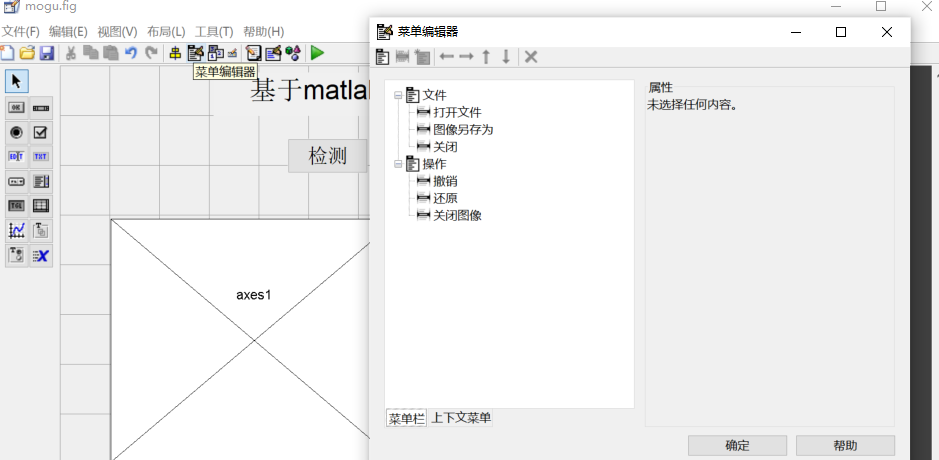将它连接到响应的回调函数或者另外写回调函数即可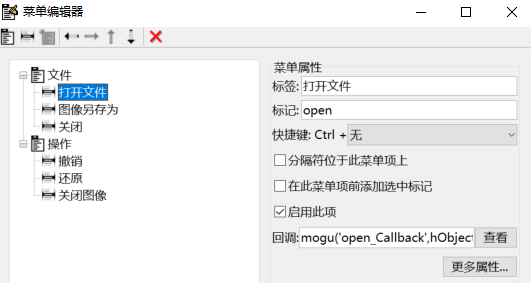## （4）成果展示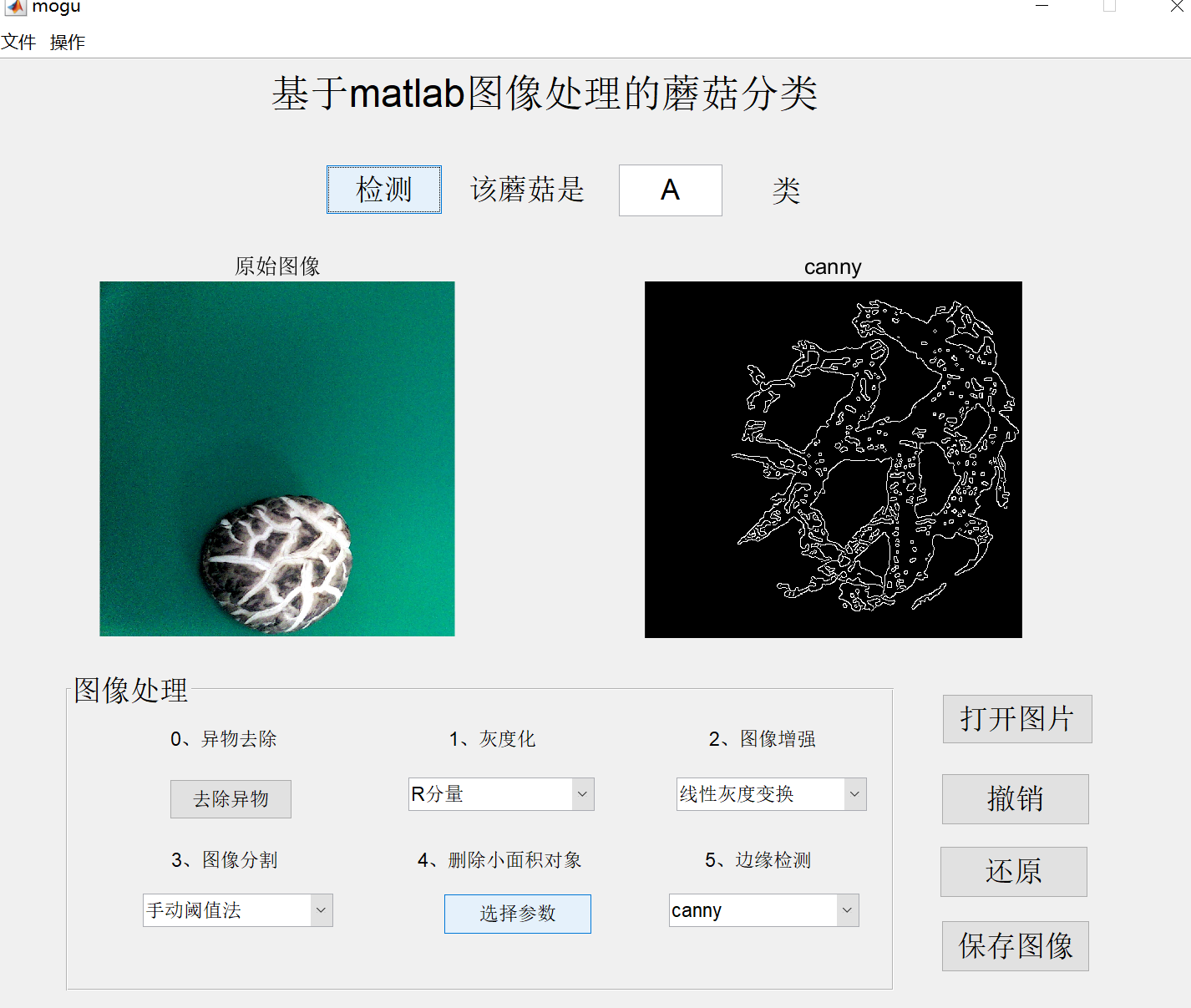展开全文开发语言
• 基于GUI搭建通用视频处理工具，matlab搭建界面，读取处理视频
• GUI界面，用于设计友好的图形用户界面，以便能为用户提供方便。
• 本人基于以前安卓手机玩猜数字的游戏的经验，基于MatlabGUI界面开发了程序，可完美实现此功能，程序包括3个文件，1个fig文件和2个m文件，可完美运行。 下面简介一下猜数字游戏的玩法： 1.点击“开始游戏”，随机...
• MATLAB交通 标志 识别，输入视频，对视频流进行识别。可制作GUI界面。可做成蓝色 ，黄色和 红色的交通标志 识别。
• 基于MATLAB-GUI图形界面的数字图像处理软件 本系统设计基于GUI图形界面，用matlab语言编写代码，实现功能包括图象的读取、存储、显示、直方图均衡化、阈值化、小波分解、小波重构、加噪、去噪、平滑、锐化、边缘检测...
• 基于MATLAB平台的PCA的人脸识别系统，可识别ORL和YALE人脸库，方法实现统一，包括GUI界面。另外可二次开发成摄像头的实时人脸系统，识别出库外人脸，可做成门禁系统，考勤系统，打卡签到系统。实现登记出勤，报警等...
• 这是一个基于matlabgui 登陆界面，简单易懂。
• 光栅衍射的GUI界面相关设计MATLAB程序代码
• Matlab界面编程GUI教程，GUI学习教程，适用于初学者
• 图形用户界面的操作步骤 目录 8.1GU设计向导 8.2编程设计GUI 83图形用户界而设计实例 习题 8.1GU设计向导 8.1.1GUI概述 8.1.2启动 GUIDE 8.1.3 GUIDE提供的控件 本节介绍: 图形用户界面(GUI)的基本概念; GUI开发...
• 如何利用MATLAB提供GUI界面实现数字图像处理系统的设计？最近无事，分享一下本科做的简单的GUI界面的图像处理系统及详细的学习笔记。本资源充分利用MATLAB GUI界面设计用户操作界面包含了基本的数字图像处理功能实现......

# matlab中gui界面设计matlab 订阅